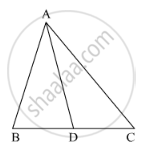Share

# In the Following Figure, Check Whether Ad is the Bisector of ∠A of ∆Abc in the Following Case: Ab = 4 Cm, Ac = 6 Cm, Bd = 1.6 Cm and Cd = 2.4 Cm - CBSE Class 10 - Mathematics

#### Question

In the following figure, check whether AD is the bisector of ∠A of ∆ABC in the following case:

AB = 4 cm, AC = 6 cm, BD = 1.6 cm and CD = 2.4 cm#### Solution

It is given that, AB = 4 cm, AC = 6 cm, BD = 1.6 cm and CD = 2.4 cm.

We have to check whether AD is bisector of ∠A.

First we will check proportional ratio between sides.

So "AB"/"AC"="BD"/"DC"

rArr4/6=1.6/2.4

rArr2/3=2/3

(It is proportional)

Hence, AD is bisector of ∠A.

Is there an error in this question or solution?

#### Video TutorialsVIEW ALL 

Solution In the Following Figure, Check Whether Ad is the Bisector of ∠A of ∆Abc in the Following Case: Ab = 4 Cm, Ac = 6 Cm, Bd = 1.6 Cm and Cd = 2.4 Cm Concept: Angle Bisector.
S